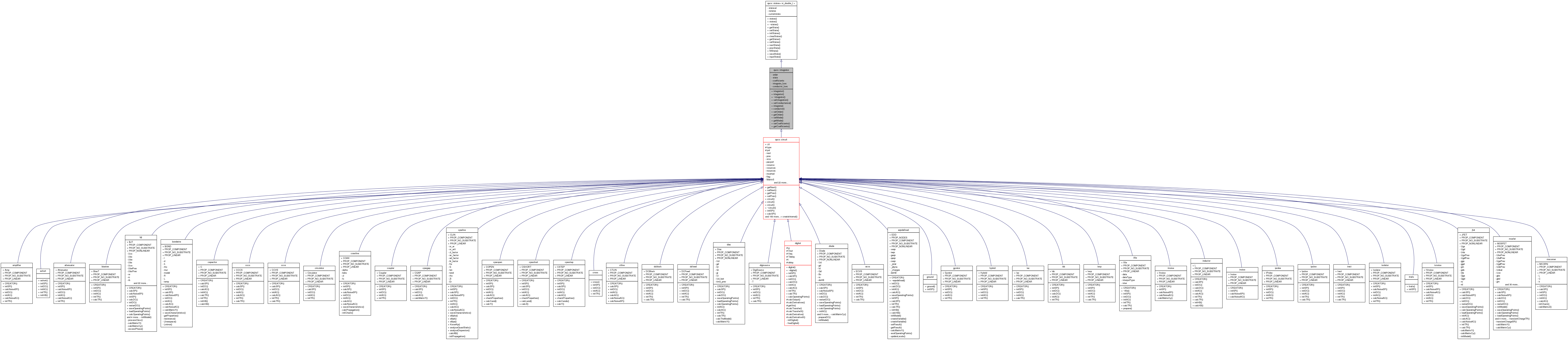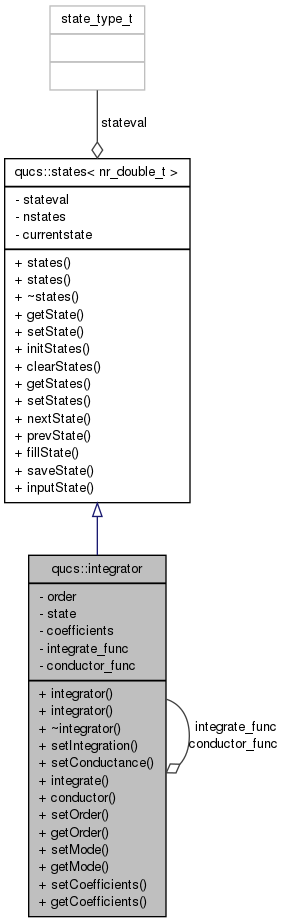Qucs-core  0.0.19
qucs::integrator Class Reference

`#include <integrator.h>`

Inheritance diagram for qucs::integrator:[legend]
Collaboration diagram for qucs::integrator:[legend]

## Public Types

typedef void(* integrate_func_t )(integrator *, int, nr_double_t, nr_double_t &, nr_double_t &)
typedef void(* conductor_func_t )(integrator *, nr_double_t, nr_double_t &)

## Public Member Functions

integrator ()
integrator (const integrator &)
~integrator ()
void setIntegration (integrate_func_t f)
void setConductance (conductor_func_t f)
void integrate (int, nr_double_t, nr_double_t &, nr_double_t &)
void conductor (nr_double_t, nr_double_t &)
void setOrder (int o)
int getOrder (void)
void setMode (int s)
int getMode (void)
void setCoefficients (nr_double_t *c)
nr_double_tgetCoefficients (void)

## Private Attributes

int order
int state
nr_double_tcoefficients
integrate_func_t integrate_func
conductor_func_t conductor_func

## Detailed Description

Definition at line 35 of file integrator.h.

## Member Typedef Documentation

 typedef void(* qucs::integrator::conductor_func_t)(integrator *, nr_double_t, nr_double_t &)

Definition at line 48 of file integrator.h.

 typedef void(* qucs::integrator::integrate_func_t)(integrator *, int, nr_double_t, nr_double_t &, nr_double_t &)

Definition at line 45 of file integrator.h.

## Constructor & Destructor Documentation

 qucs::integrator::integrator ( )

Definition at line 43 of file integrator.cpp.

 qucs::integrator::integrator ( const integrator & c )

Definition at line 53 of file integrator.cpp.

 qucs::integrator::~integrator ( )

Definition at line 62 of file integrator.cpp.

## Member Function Documentation

 void qucs::integrator::conductor ( nr_double_t cap, nr_double_t & geq )

Definition at line 76 of file integrator.cpp.

 nr_double_t* qucs::integrator::getCoefficients ( void ) ` [inline]`

Definition at line 57 of file integrator.h.

 int qucs::integrator::getMode ( void ) ` [inline]`

Definition at line 55 of file integrator.h.

 int qucs::integrator::getOrder ( void ) ` [inline]`

Definition at line 53 of file integrator.h.

 void qucs::integrator::integrate ( int qstate, nr_double_t cap, nr_double_t & geq, nr_double_t & ceq )

Definition at line 67 of file integrator.cpp.

 void qucs::integrator::setCoefficients ( nr_double_t * c ) ` [inline]`

Definition at line 56 of file integrator.h.

 void qucs::integrator::setConductance ( conductor_func_t f ) ` [inline]`

Definition at line 49 of file integrator.h.

 void qucs::integrator::setIntegration ( integrate_func_t f ) ` [inline]`

Definition at line 46 of file integrator.h.

 void qucs::integrator::setMode ( int s ) ` [inline]`

Definition at line 54 of file integrator.h.

 void qucs::integrator::setOrder ( int o ) ` [inline]`

Definition at line 52 of file integrator.h.

## Field Documentation

 nr_double_t* qucs::integrator::coefficients` [private]`

Definition at line 62 of file integrator.h.

 conductor_func_t qucs::integrator::conductor_func` [private]`

Definition at line 64 of file integrator.h.

 integrate_func_t qucs::integrator::integrate_func` [private]`

Definition at line 63 of file integrator.h.

 int qucs::integrator::order` [private]`

Definition at line 60 of file integrator.h.

 int qucs::integrator::state` [private]`

Reimplemented in relais.

Definition at line 61 of file integrator.h.

The documentation for this class was generated from the following files: